##### Chemistry Workbook For Dummies with Online Practice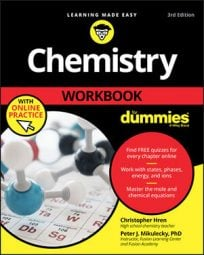A conversion factor uses your knowledge of the relationships between units to convert from one unit to another. For example, if you know that there are 2.54 centimeters in every inch (or 2.2 pounds in every kilogram or 101.3 kilopascals in every atmosphere), then converting between those units becomes simple algebra. It is important to know some common conversions of temperature, size, and pressure as well as metric prefixes.

## Conversion factor table

The following table includes some useful conversion factors.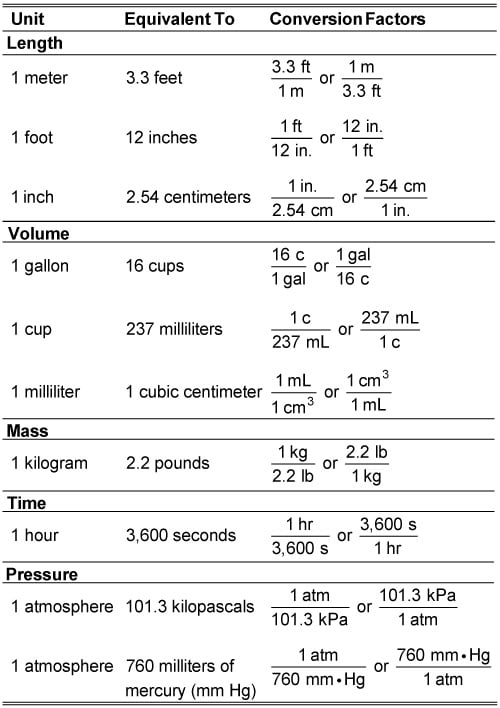## Using conversion factors example

The following example shows how to use a basic conversion factor to fix non-SI units.

Dr. Geekmajor absentmindedly measures the mass of a sample to be 0.75 lb and records his measurement in his lab notebook. His astute lab assistant, who wants to save the doctor some embarrassment, knows that there are 2.2 lbs in every kilogram. The assistant quickly converts the doctor’s measurement to SI units. What does she get?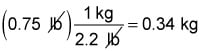Let’s try another example. A chemistry student, daydreaming during lab, suddenly looks down to find that he’s measured the volume of his sample to be 1.5 cubic inches. What does he get when he converts this quantity to cubic centimeters?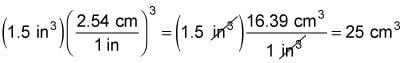Rookie chemists often mistakenly assume that if there are 2.54 centimeters in every inch, then there are 2.54 cubic centimeters in every cubic inch. No! Although this assumption seems logical at first glance, it leads to catastrophically wrong answers. Remember that cubic units are units of volume and that the formula for volume is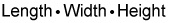Imagine 1 cubic inch as a cube with 1-inch sides. The cube’s volume is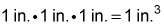Now consider the dimensions of the cube in centimeters: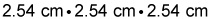Calculate the volume using these measurements, and you get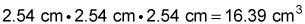This volume is much greater than 2.54 cm3! To convert units of area or volume using length measurements, square or cube everything in your conversion factor, not just the units, and everything works out just fine.